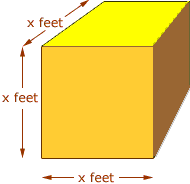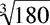Quandaries and Queries Hello,   I have one for you that's got me stumped. Is it possible to calculate the dimensions of a square if I have the cubic feet? Say I know the package to be 180 cubic feet.   Thank you,   Jake Hi Jake, Since you use the units cubic feet I expect that you hae a cube rather than a square.A cube x feet by x feet by x feet has volume x3 cubic feet. Hence in your example you have x3 = 180 cubic feet and you want to find x. x is the cube root of 180, ie x =. There is probably a cube root button on your calculator. On mine I found that the cube root of 180 is approximately 5.646. Hence a cube with volume 180 cubic feet is approximately 5.646 feet on each side. Penny Go to Math Central